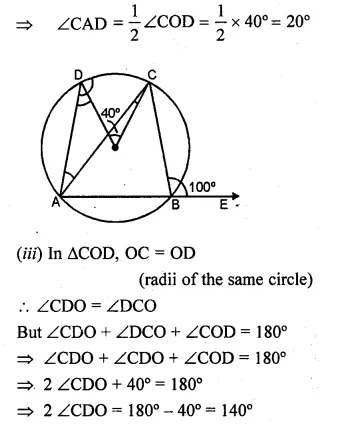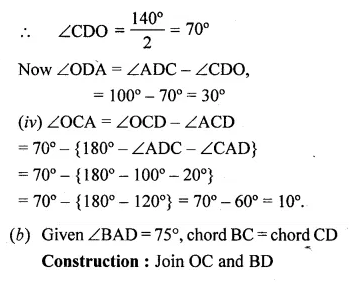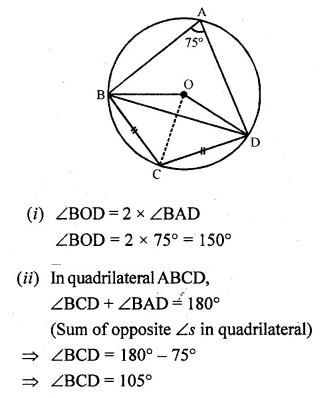Guru

# Question 11. (a) In the figure (i) given below, O is the centre of the circle. If ∠COD = 40° and ∠CBE = 100°, then find : (i) ∠ADC (ii) ∠DAC (iii) ∠ODA (iv) ∠OCA. (b) In the figure (ii) given below, O is the centre of the circle. If ∠BAD = 75° and BC = CD, find : (i) ∠BOD (ii) ∠BCD (iii) ∠BOC (iv) ∠OBD

• 3

This is an important and exam oriented question from Chapter name- circles
Topic – Angle properties of circles
Chapter number- 15. This question has been asked in previous year question paper of 2009

In the figure(i) given, O is the centre of the circle. ∠COD = 40° and ∠CBE = 100°, then we have to find : (i) ∠ADC (ii) ∠DAC (iii) ∠ODA (iv) ∠OCA.

In the figure (ii) given  O is the centre of the circle.  ∠BAD = 75° and BC = CD,

Now we have to find : (i) ∠BOD (ii) ∠BCD (iii) ∠BOC (iv) ∠OBD

ICSE Avichal publication
Understanding ICSE Mathematics
Question 11

Share

1. Solution:
(a) (i) ∴ ABCD is a cyclic quadrilateral.